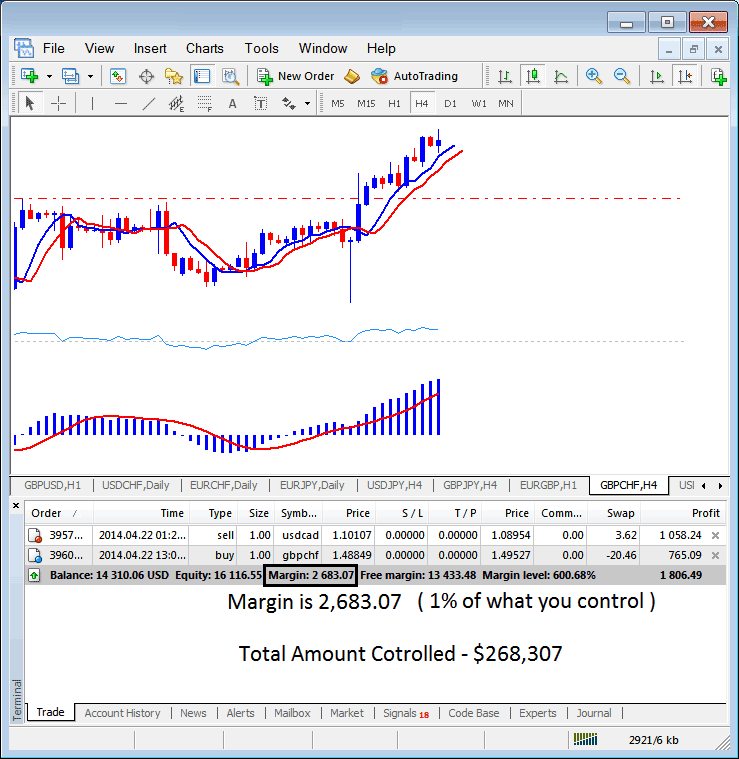Margin required in this case is 1,000 dollars (your money) if it is expressed as a percent of 100,000 dollars which you control it is:

If indices trading leverage = 100:1

1,000 / 100,000 * 100= 1%

Margin required = 1%

(1/100 *100= 1%)

(Simplify - your indices trading capital is \$1,000 after stock indices leverage you control \$100,000 - \$1,000 is what percent of \$100,000 - it is 1%) that is your indices trading margin requirement for your stock indexes trading account.

The indices trading margin example explained and illustrated below, the set stock indices leverage is 100:1, the indices trading margin which is 1% is \$2683.07, therefore the total amount controlled by the indices trader is: \$268,307 - this is because with this indices trading leverage the indices trader has used little of his money and borrowed the rest, with this set at 100:1, the indices trader is using 1 % of their trading capital, this 1% is \$2683.07, if 1% is \$2683.07 then 100% is \$268,307• If = 50:1 Indices Trading Leverage - Used Indices Trading Leverage

Then indices trading margin requirement = 1/50 *100= 2%

if you have \$1,000,

1,000* 50 = \$50,000.

1,000 / 50,000 * 100= 2%

(Simplify - your indices trading capital is \$1,000 after stock indices leverage you control \$50,000 - \$1,000 is what percent of \$50,000 - it is 2%) that is your indices trading margin requirement

• If = 20:1 Indices Trading Leverage - Used Indices Trading Leverage

Then the indices trading margin requirement = 1/20 *100= 5%

if you have \$1,000,

1,000* 20 = \$20,000.

1,000 / 20,000 * 100= 5%

(Simplify - your indices trading capital is \$1,000 after stock indices leverage you control \$20,000 - \$1,000 is what percent of \$20,000 - it is 5%) that is your indices trading margin requirement

• If = 10:1 Indices Trading Leverage - Used Indices Trading Leverage

Then the indices trading margin requirement is = 1/10 *100= 10%

if you have \$1,000,

1,000* 10 = \$10,000.

1,000 / 10,000 * 100= 10%

(Simplify - your indices trading capital is \$1,000 after stock indices leverage you control \$10,000 - \$1,000 is what percent of \$10,000 - it is 10%) that is your indices trading margin requirement

### What is The Difference Between Maximum Indices Trading Leverage and Used Indices Trading Leverage

If your indices broker has given you 100:1 Maximum Indices Trading Leverage, but you only open a trade of 10,000 dollars then Used Indices Trading Leverage is:

10,000 dollars: 1,000 dollars (your money)

10:1

In the above MetaTrader 4 indices trading screenshot example, the indices trader is using \$2683.07, the total controlled amount is \$268,307, but indices trading account equity is \$16,116.55, therefore used stock indices leverage is (\$268,307 divide by 16,116.55) = 16.64 : 1

16.64 : 1 Used Indices Trading Leverage

Indices Trading Margin accounts allows traders to control a large amounts of indices trading units using leverage using little of their own capital while borrowing the rest

Obtaining this indices trading account will enable you to borrow money from the indices broker to trade indices lots with.

The amount of borrowing power your account gives you what is called “ indices trading leverage”, and is usually expressed as a ratio - a ratio of 100:1 means you can control resources worth 100 times your deposit.

What this means in Indices Trading terms is that with 1% margin in your indices trading account you can control a trade worth \$100,000 with a \$1,000 deposit.

However, Trading this margin indices trading account increases both potential for profits as well as losses. In Indices Trading you can never lose more than you invest, losses are limited to your deposits and usually brokers will close a transaction that extends beyond your deposit amount by executing a margin call. Indices traders must therefore try to keep their margin level above that required. By using indices trading money management rules and keeping your used indices trading leverage below 5:1.

Start Trading Crypto CFDs with a \$30 Bonus